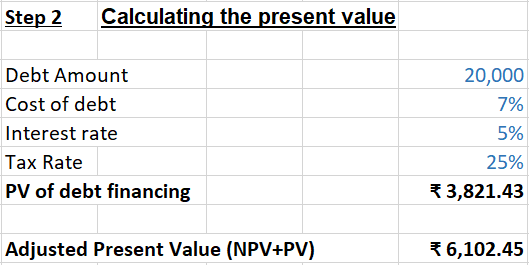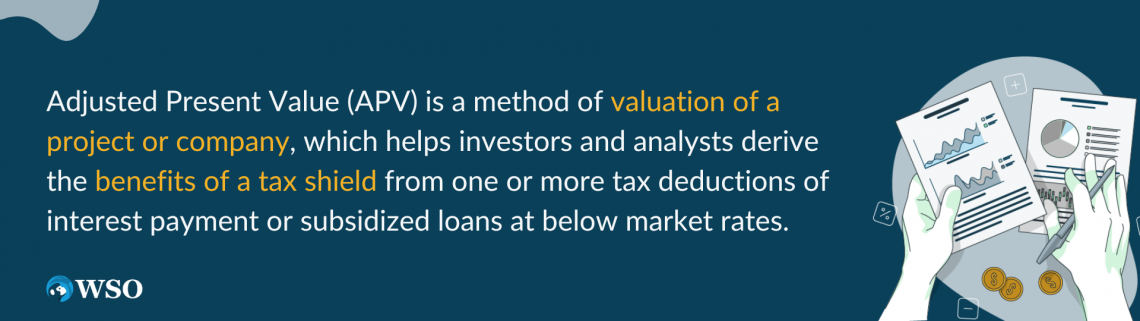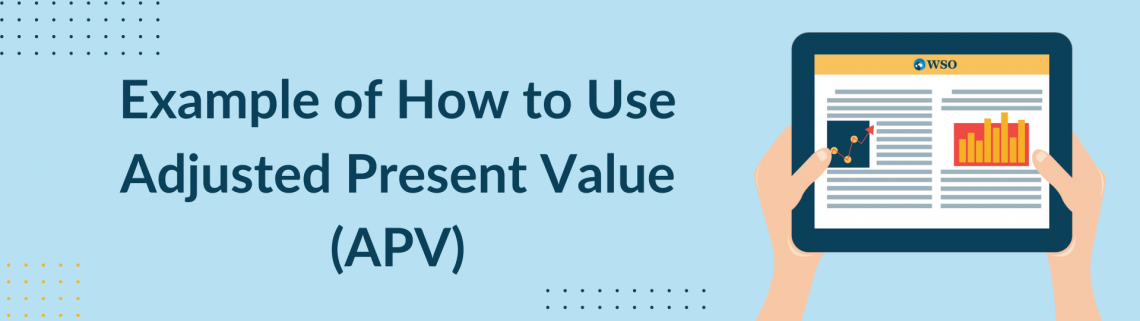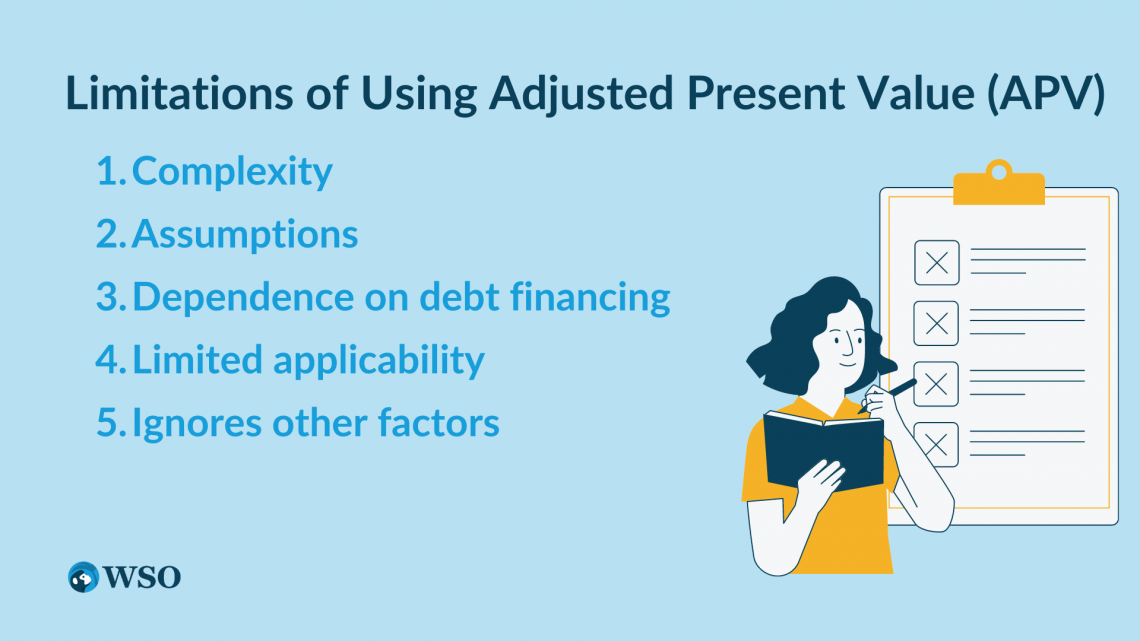Valuation method that calculates the present value of a project or investment as if it were all-equity financed, using the Net Present Value method

Adjusted Present Value (APV) is used to evaluate projects and companies. It is a valuation method introduced by Stewart Myers in 1974.It takes the net present value (NPV) of the company or the project, assuming it is financed solely by equity, and adds the present value of debt financing costs, which include interest tax shields, costs of debt issuance, costs of financial distress, financial subsidies, etc...

The basic idea behind APV is, first, to calculate the value of a project or investment as if it were all-equity financed, using the Net Present Value (NPV) method. This calculation provides the project or investment's base value, assuming there is no debt financing.

The value of tax shields or other financial benefits from debt financing is calculated separately and added to the base value. This adjustment accounts for the tax benefits that arise from using debt financing.

To calculate APV, we use the following formula:

Adjusted Present Value = Unlevered Firm Value + Net Effect of debt

The net effect of debt includes the tax benefits that are created when the interest on a company's debt is tax-deductible. The tax benefit is determined by multiplying the interest expense by the tax rate, and it only applies to the interest and tax paid in a given year.

To determine the present value of the interest tax shield, one can calculate (the tax rate multiplied by the debt load multiplied by the interest rate) divided by the interest rate.

## ​How to Calculate Adjusted Present Value (APV)?

Calculating APV involves calculations for different components altogether. A positive APV is always favored compared to a negative APV. One must follow a few structured steps, which must be followed in sequence.These steps are as mentioned below:

1. Find the value of the un-levered firms
An un-levered firm is a company that has no debt in its capital structure

In other words, it is a firm that is entirely financed by equity. It is the most important element of calculating the value. It includes the cost of equity, the expected future capital structure, and the expected future free cash flow.

2. Calculate the net value of debt financing

This includes multiple elements. It is the sum of various effects altogether, which includes elements such as follow:

• PV(interest tax shields) – our main focus
• PV(issuance costs)
• PV(financial distress costs)
• PV(other market imperfections)

3. Calculate the total

Finally, calculate the value of the un-levered project or company and the net value of the debt financing to find out the APV of the project.

If the calculation is difficult from the un-levered side of the cost of equity, then one can opt for the levered side of the approach. And if the tax shield discount rate is unknown in the calculation, then one can use a larger or equal rate to the cost of debt and less than or equal to the un-levered cost of equity.

## How to Calculate APV in Excel?

Excel can be used to calculate APV more efficiently and effectively. Calculating the APV involves several structured steps, which are mentioned below:Step 1 - Begin with the Calculation of the NPV of cash flows, assuming that the finance is all-equity financing:

to the last year of the project or investment (to the period it is continued).

Then Calculate the discount rate (r) of the company based on the company's cost of equity or the weighted average cost of capital (WACC).

While using the Excel function of Net present value (NPV) to calculate the present value of the cash flows of the company:

=NPV(r, cash flows)Step 2 - Calculate the present value by adding the debt financing amount to the NPV:

We should determine the tax rate which applies to the company according to the country in which it is registered.

Then one should calculate the present value of the tax shield using the following formula:

PV = Tax Rate * Present Value of Debt Tax Shield

Finally, add the present value of the tax shield to the Net Present Value NPV of discounted cash flows of the company, which was derived earlier to obtain the APV as follows:

APV = NPV + PV## What Does Adjusted Present Value Tell You?

APV is a method of valuation of a project or company, which helps investors and analysts derive the benefits of a tax shield from one or more tax deductions of interest payment or subsidized loans at below-market rates.APV is a preferred tool among investors while calculating leveraged transactions or the value of a project financed with equity because leveraged buyout situations are mainly the most effective situation with this valuation methodology.

This approach is used mostly when other traditional valuation methods, like discounted cash flow, may not present the accurate value of a company or investment in the project because of the presence of factors such as non-operating assets, tax shields available, and other factors that are sometimes hidden in traditional valuation methods.

The cost of capital tends to fall when leverage is used due to the value of a debt-financed project can be higher than just an equity-financed project. The use of debt on the capital structure can turn a negative NPV project into a positive one.

## NOTE

Net Present value (NPV) uses the weighted average cost of capital as the discount rate, while APV uses the cost of equity as the discount rate.

With the use of the APV method, investors and analysts can have a more accurate and true value of that particular company or investment in the project, making them make better-informed decisions regarding their investment in a particular opportunity.

## Example of How to Use Adjusted Present Value (APV)

While calculating the projection through the APV, the NPV is also calculated, and the sum of the present value of the interest tax shield is added to obtain the adjusted present value.Investors calculate the APV by first adding the present value of a company's operating assets to the present value of any financing side effects.

And APV values a company by first determining the value of the project or company if it were to be financed entirely by equity without any debt, then adding the value of the tax shield that comes with taking on debt to the equity value.

The tax shield given above represents the amount of money that the company saves on taxes by deducting interest payments from its taxable net income.

Taking a hypothetical example, a multi-year projection calculation shows that the present value of the company XYZ's free cash flow, including terminal value, is $500,000, with the tax rate being 30% and interest rates standing at 7%.$100,000 debt load has an interest tax shield of $30,000, which is calculated as: ($100,000 * 30% * 7%) / 7%

This leaves us with a present value of ($500,000 +$30,000) = \$530,000.

This way, investors and analysts can find a project or company's adjusted present value as required.

## The Difference Between APV and DCF

APV and DCF are two different methods that are used in corporate finance for estimating the value of a company or project.Here are some of their main differences:

APV Vs. DCF

BasisAdjusted Present Value (APV)Discounted Cash Flow (DCF)
ConceptFairly similar to DCF in its methodology and purpose but does not use WACC.The Discounted Cash Flow (DCF) uses the weighted average cost of capital to calculate the APV with the effect of the cost of equity and cost of debt separately.
MethodologyAPV methodology does not capture taxes or other financial effects such as weighted average cost of capital WACC or other financial discount rates.This method captures the financial effects and taxes as the weighted average cost of capital WACC and other financial discount rates.
Complexity It can be a bit more complex while calculating due to needing to calculate separate discount rates for different components of a company or a project. It is a simpler method as it only requires a single discount rate to complete its calculations.
ApplicabilityIt is used in very specific situations, which are the valuation of highly leveraged companies or with significant tax implications.DCF is a more widely used method for the valuation of companies or projects in various industries.

## Limitations of Using Adjusted Present Value (APV)

In general, the APV is not used as often as the discounted cash flow or as any other method because it is more of an academic calculation but is often considered to result in more accurate valuations.Some of the limitations are mentioned below:

1. Complexity
The APV model can be a bit more complex than other valuation methods, as it requires multiple calculations to determine the present value of the tax shield.

2. Assumptions
APV is based on numerous assumptions, including the cost of debt and the tax rate. If these assumptions are inaccurate or change, the valuation may be misleading.

3. Dependence on debt financing
APV assumes that debt financing is available and can be used to increase the company's value. However, in some cases where a company may not be able to obtain debt financing or may be highly leveraged, this can limit the effectiveness of the APV model.

4. Limited applicability
APV is most useful for valuing companies with predictable cash flows and stable debt levels. It may be less effective for valuing companies with fluctuating cash flows or high debt levels.

5. Ignores other factors
APV focuses mostly on the tax benefits of debt financing and does not consider other factors that can also impact a company's value, such as market conditions, competition, or technological change, which are also critical factors.

## Key Takeaways

• Adjusted present value (APV) is the net present value of a company or project, assuming it is solely financed by equity, plus the present value of financing benefits.
• APV helps the investor to understand the benefits of tax shields from interest payments.
• This method is best used for transactions that are leveraged, such as leveraged buyouts, but is more of an academic calculation.
• APV is particularly useful for valuing projects with complex financing structures, such as those involving multiple funding sources or different types of debt.
• The key advantages of using APV over other valuation methods include its ability to incorporate the effects of financing costs and its flexibility in handling complex financing structures.### Everything You Need To Master Valuation Modeling

To Help You Thrive in the Most Prestigious Jobs on Wall Street.

Researched and authored by Ashish Jangra | Linkedin

Reviewed and Edited by Wissam El Maouch | LinkedIn